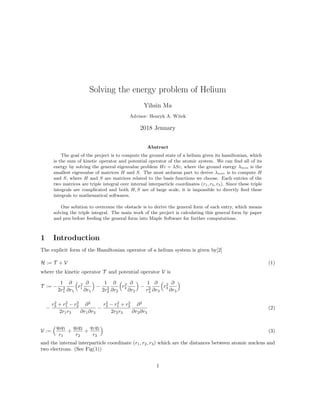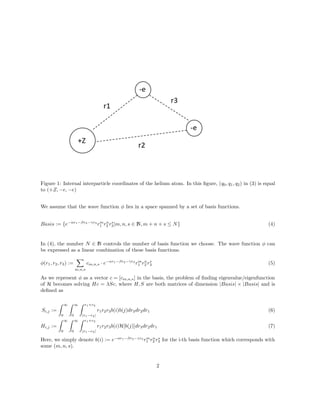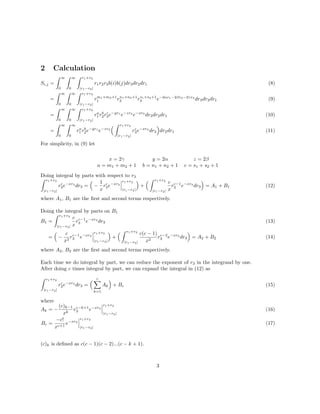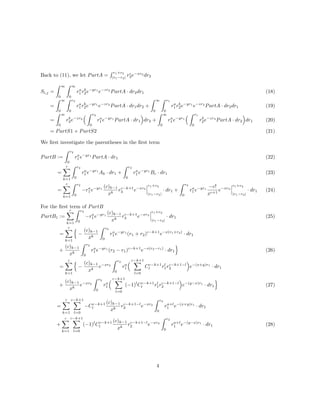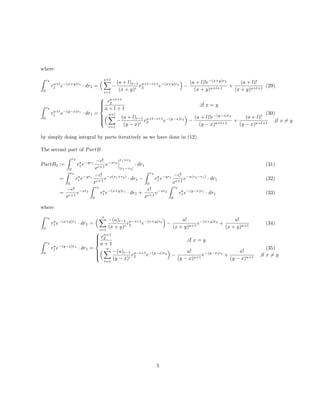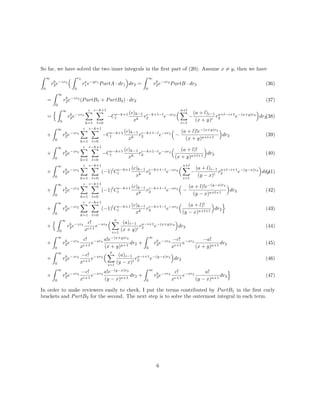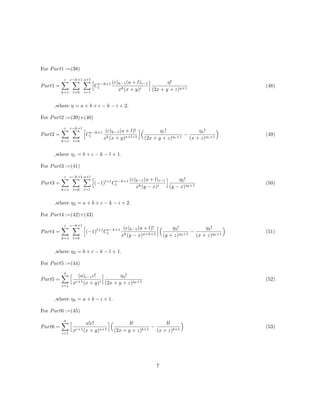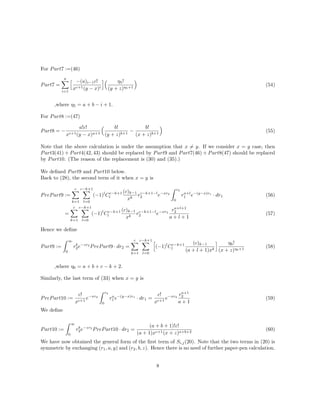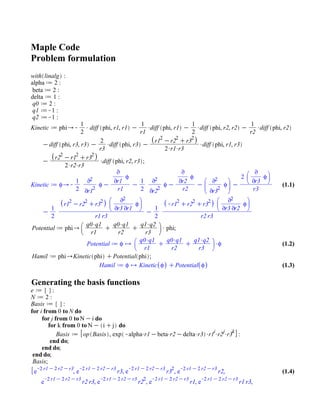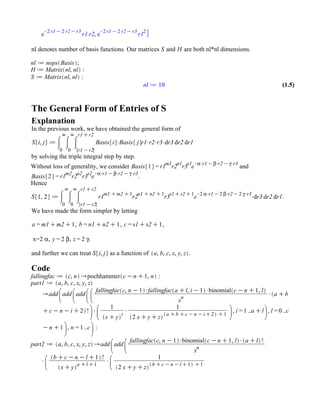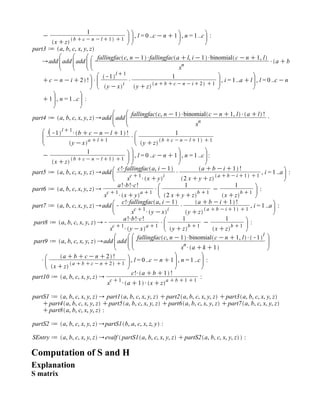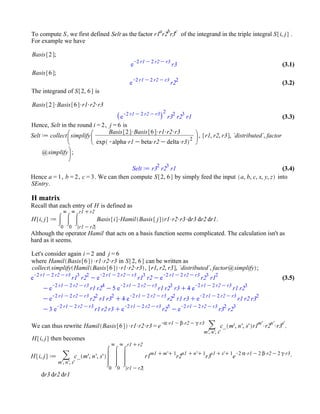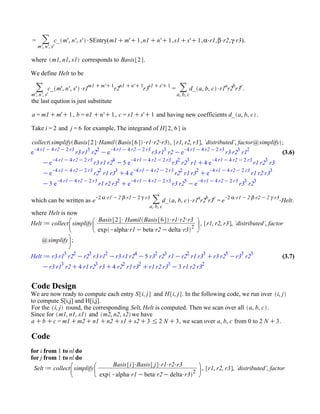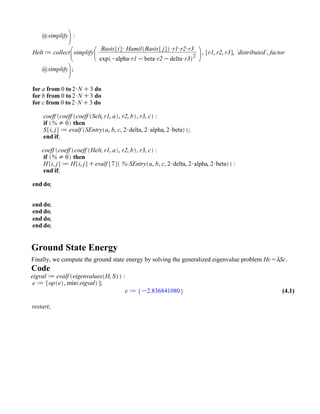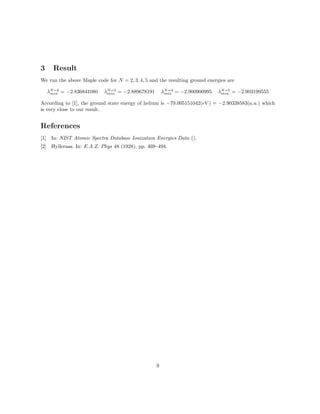Próximo SlideShare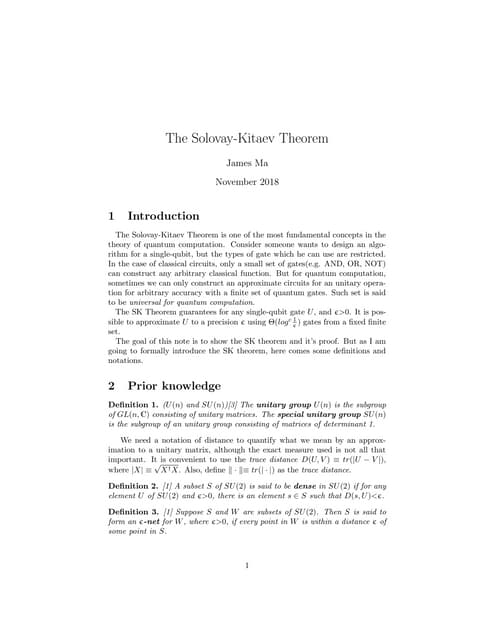Solovay Kitaev theorem
Cargando en ... 3
1 de 15

### Solving the energy problem of helium final report

1. Solving the energy problem of Helium Yihsin Ma Advisor: Henryk A. Witek 2018 Jenuary Abstract The goal of the project is to compute the ground state of a helium given its hamiltonian, which is the sum of kinetic operator and potential operator of the atomic system. We can ﬁnd all of its energy by solving the general eigenvalue problem Hc = λSc, where the ground energy λmin is the smallest eigenvalue of matrices H and S. The most arduous part to derive λmin is to compute H and S, where H and S are matrices related to the basis functions we choose. Each entries of the two matrices are triple integral over internal interparticle coordinates (r1, r2, r3). Since these triple integrals are complicated and both H, S are of large scale, it is impossible to directly feed these integrals to mathematical softwares. One solution to overcome the obstacle is to derive the general form of each entry, which means solving the triple integral. The main work of the project is calculating this general form by paper and pen before feeding the general form into Maple Software for further computations. 1 Introduction The explicit form of the Hamiltonian operator of a helium system is given by H := T + V (1) where the kinetic operator T and potential operator V is T := − 1 2r2 1 ∂ ∂r1 r2 1 ∂ ∂r1 − 1 2r2 2 ∂ ∂r2 r2 2 ∂ ∂r2 − 1 r2 3 ∂ ∂r3 r2 3 ∂ ∂r3 − r2 3 + r2 1 − r2 2 2r1r3 ∂2 ∂r1∂r3 − r2 3 − r2 1 + r2 2 2r2r3 ∂2 ∂r2∂r3 (2) V := q0q1 r1 + q0q2 r2 + q1q2 r3 (3) and the internal interparticle coordinate (r1, r2, r3) which are the distances between atomic nucleus and two electrons. (See Fig(1)) 1
2. Figure 1: Internal interparticle coordinates of the helium atom. In this ﬁgure, (q0, q1, q2) in (3) is equal to (+Z, −e, −e) We assume that the wave function φ lies in a space spanned by a set of basis functions. Basis := {e−αr1−βr2−γr3 rm 1 rn 2 rs 3|m, n, s ∈ N, m + n + s ≤ N} (4) In (4), the number N ∈ N controls the number of basis function we choose. The wave function φ can be expressed as a linear combination of these basis functions. φ(r1, r2, r3) := m,n,s cm,n,s · e−αr1−βr2−γr3 rm 1 rn 2 rs 3 (5) As we represent φ as a vector c = [cm,n,s] in the basis, the problem of ﬁnding eigenvalue/eigenfunction of H becomes solving Hc = λSc, where H, S are both matrices of dimension |Basis| × |Basis| and is deﬁned as Si,j := ∞ 0 ∞ 0 r1+r2 |r1−r2| r1r2r3b(i)b(j)dr3dr2dr1 Hi,j := ∞ 0 ∞ 0 r1+r2 |r1−r2| r1r2r3b(i)H[b(j)]dr3dr2dr1 (6) (7) Here, we simply denote b(i) := e−αr1−βr2−γr3 rm 1 rn 2 rs 3 for the i-th basis function which corresponds with some (m, n, s). 2
3. 2 Calculation Si,j = ∞ 0 ∞ 0 r1+r2 |r1−r2| r1r2r3b(i)b(j)dr3dr2dr1 = ∞ 0 ∞ 0 r1+r2 |r1−r2| rm1+m2+1 1 rn1+n2+1 2 rs1+s2+1 3 e−2αr1−2βr2−2γr3 dr3dr2dr1 = ∞ 0 ∞ 0 r1+r2 |r1−r2| ra 1 rb 2rc 3e−yr1 e−zr2 e−xr3 dr3dr2dr1 = ∞ 0 ∞ 0 ra 1 rb 2e−yr1 e−zr2 r1+r2 |r1−r2| rc 3e−xr3 dr3 dr2dr1 (8) (9) (10) (11) For simplicity, in (9) let x = 2γ y = 2α z = 2β a = m1 + m2 + 1 b = n1 + n2 + 1 c = s1 + s2 + 1 Doing integral by parts with respect to r3 r1+r2 |r1−r2| rc 3e−xr3 dr3 = − 1 x rc 3e−xr3 r1+r2 |r1−r2| + r1+r2 |r1−r2| c x rc−1 3 e−xr3 dr3 = A1 + B1 (12) where A1, B1 are the ﬁrst and second terms respectively. Doing the integral by parts on B1 B1 = r1+r2 |r1−r2| c x rc−1 3 e−xr3 dr3 = − c x2 rc−1 3 e−xr3 r1+r2 |r1−r2| + r1+r2 |r1−r2| c(c − 1) x2 rc−2 3 e−xr3 dr3 = A2 + B2 (13) (14) where A2, B2 are the ﬁrst and second terms respectively. Each time we do integral by part, we can reduce the exponent of r3 in the integrand by one. After doing c times integral by part, we can expand the integral in (12) as r1+r2 |r1−r2| rc 3e−xr3 dr3 = c k=1 Ak + Bc (15) where Ak = − (c)k−1 xk rc−k+1 3 e−xr3 r1+r2 |r1−r2| Bc = −c! xc+1 e−xr3 r1+r2 |r1−r2| (16) (17) (c)k is deﬁned as c(c − 1)(c − 2)...(c − k + 1). 3
4. Back to (11), we let PartA = r1+r2 |r1−r2| rc 3e−xr3 dr3 Si,j = ∞ 0 ∞ 0 ra 1 rb 2e−yr1 e−zr2 PartA · dr2dr1 = ∞ 0 r2 0 ra 1 rb 2e−yr1 e−zr2 PartA · dr1dr2 + ∞ 0 r1 0 ra 1 rb 2e−yr1 e−zr2 PartA · dr2dr1 = ∞ 0 rb 2e−zr2 r2 0 ra 1 e−yr1 PartA · dr1 dr2 + ∞ 0 ra 1 e−yr1 r1 0 rb 2e−zr2 PartA · dr2 dr1 = PartS1 + PartS2 (18) (19) (20) (21) We ﬁrst investigate the parentheses in the ﬁrst term PartB := r2 0 ra 1 e−yr1 PartA · dr1 = c k=1 r2 0 ra 1 e−yr1 Ak · dr1 + r2 0 ra 1 e−yr1 Bc · dr1 = c k=1 r2 0 −ra 1 e−yr1 (c)k−1 xk rc−k+1 3 e−xr3 r1+r2 |r1−r2| · dr1 + r2 0 ra 1 e−yr1 −c! xc+1 e−xr3 r1+r2 |r1−r2| · dr1 (22) (23) (24) For the ﬁrst term of PartB PartB1 := c k=1 r2 0 −ra 1 e−yr1 (c)k−1 xk rc−k+1 3 e−xr3 r1+r2 |r1−r2| · dr1 = c k=1 − (c)k−1 xk r2 0 ra 1 e−yr1 (r1 + r2)c−k+1 e−x(r1+r2) · dr1 + (c)k−1 xk r2 0 ra 1 e−yr1 (r2 − r1)c−k+1 e−x(r2−r1) · dr1 = c k=1 − (c)k−1 xk e−xr2 r2 0 ra 1 c−k+1 l=0 Cc−k+1 l rl 1rc−k+1−l 2 e−(x+y)r1 · dr1 + (c)k−1 xk e−xr2 r2 0 ra 1 c−k+1 l=0 (−1)l Cc−k+1 l rl 1rc−k+1−l 2 e−(y−x)r1 · dr1 (25) (26) (27) = c k=1 c−k+1 l=0 −Cc−k+1 l (c)k−1 xk rc−k+1−l 2 e−xr2 r2 0 ra+l 1 e−(x+y)r1 · dr1 + c k=1 c−k+1 l=0 (−1)l Cc−k+1 l (c)k−1 xk rc−k+1−l 2 e−xr2 r2 0 ra+l 1 e−(y−x)r1 · dr1 (28) 4
5. where r2 0 ra+l 1 e−(x+y)r1 · dr1 = a+l i=1 − (a + l)i−1 (x + y)i ra+l−i+1 2 e−(x+y)r2 − (a + l)!e−(x+y)r2 (x + y)a+l+1 + (a + l)! (x + y)a+l+1 (29) r2 0 ra+l 1 e−(y−x)r1 · dr1 =    ra+l+1 2 a + l + 1 ,if x = y a+l i=1 − (a + l)i−1 (y − x)i ra+l−i+1 2 e−(y−x)r2 − (a + l)!e−(y−x)r2 (y − x)a+l+1 + (a + l)! (y − x)a+l+1 ,if x = y (30) by simply doing integral by parts iteratively as we have done in (12). The second part of PartB PartB2 := r2 0 ra 1 e−yr1 −c! xc+1 e−xr3 r1+r2 |r1−r2| · dr1 = r2 0 ra 1 e−yr1 −c! xc+1 e−x(r1+r2) · dr1 − r2 0 ra 1 e−yr1 −c! xc+1 e−x(r2−r1) · dr1 = −c! xc+1 e−xr2 r2 0 ra 1 e−(x+y)r1 · dr1 + c! xc+1 e−xr2 r2 0 ra 1 e−(y−x)r1 · dr1 (31) (32) (33) where r2 0 ra 1 e−(x+y)r1 · dr1 = a i=1 −(a)i−1 (x + y)i ra−i+1 2 e−(x+y)r2 − a! (x + y)a+1 e−(x+y)r2 + a! (x + y)a+1 r2 0 ra 1 e−(y−x)r1 · dr1 =    ra+1 2 a + 1 ,if x = y a i=1 −(a)i−1 (y − x)i ra−i+1 2 e−(y−x)r2 − a! (y − x)a+1 e−(y−x)r2 + a! (y − x)a+1 ,if x = y (34) (35) 5
6. So far, we have solved the two inner integrals in the ﬁrst part of (20). Assume x = y, then we have ∞ 0 rb 2e−zr2 r2 0 ra 1 e−yr1 PartA · dr1 dr2 = ∞ 0 rb 2e−zr2 PartB · dr2 (36) = ∞ 0 rb 2e−zr2 (PartB1 + PartB2) · dr2 = ∞ 0 rb 2e−zr2 c k=1 c−k+1 l=0 −Cc−k+1 l (c)k−1 xk rc−k+1−l 2 e−xr2 a+l i=1 − (a + l)i−1 (x + y)i ra+l−i+1 2 e−(x+y)r2 dr2 + ∞ 0 rb 2e−zr2 c k=1 c−k+1 l=0 −Cc−k+1 l (c)k−1 xk rc−k+1−l 2 e−xr2 − (a + l)!e−(x+y)r2 (x + y)a+l+1 dr2 + ∞ 0 rb 2e−zr2 c k=1 c−k+1 l=0 −Cc−k+1 l (c)k−1 xk rc−k+1−l 2 e−xr2 (a + l)! (x + y)a+l+1 dr2 + ∞ 0 rb 2e−zr2 c k=1 c−k+1 l=0 (−1)l Cc−k+1 l (c)k−1 xk rc−k+1−l 2 e−xr2 a+l i=1 − (a + l)i−1 (y − x)i ra+l−i+1 2 e−(y−x)r2 dr2 + ∞ 0 rb 2e−zr2 c k=1 c−k+1 l=0 (−1)l Cc−k+1 l (c)k−1 xk rc−k+1−l 2 e−xr2 − (a + l)!e−(y−x)r2 (y − x)a+l+1 dr2 + ∞ 0 rb 2e−zr2 c k=1 c−k+1 l=0 (−1)l Cc−k+1 l (c)k−1 xk rc−k+1−l 2 e−xr2 (a + l)! (y − x)a+l+1 dr2 + ∞ 0 rb 2e−zr2 c! xc+1 e−xr2 a i=1 (a)i−1 (x + y)i ra−i+1 2 e−(x+y)r2 dr2 + ∞ 0 rb 2e−zr2 c! xc+1 e−xr2 a!e−(x+y)r2 (x + y)a+1 dr2 + ∞ 0 rb 2e−zr2 −c! xc+1 e−xr2 −a! (x + y)a+1 dr2 + ∞ 0 rb 2e−zr2 −c! xc+1 e−xr2 a i=1 (a)i−1 (y − x)i ra−i+1 2 e−(y−x)r2 dr2 + ∞ 0 rb 2e−zr2 −c! xc+1 e−xr2 a!e−(y−x)r2 (y − x)a+1 dr2 + ∞ 0 rb 2e−zr2 c! xc+1 e−xr2 a! (y − x)a+1 dr2 (37) (38) (39) (40) (41) (42) (43) (44) (45) (46) (47) In order to make reviewers easily to check, I put the terms contributed by PartB1 in the ﬁrst curly brackets and PartB2 for the second. The next step is to solve the outermost integral in each term. 6
7. For Part1 :=(38) Part1 = c k=1 c−k+1 l=0 a+l i=1 Cc−k+1 l (c)k−1(a + l)i−1 xk(x + y)i η! (2x + y + z)η+1 ,where η = a + b + c − k − i + 2. (48) For Part2 :=(39)+(40) Part2 = c k=1 c−k+1 l=0 Cc−k+1 l (c)k−1(a + l)! xk(x + y)a+l+1 η1! (2x + y + z)η1+1 − η1! (x + z)η1+1 ,where η1 = b + c − k − l + 1. (49) For Part3 :=(41) Part3 = c k=1 c−k+1 l=0 a+l i=1 (−1)l+1 Cc−k+1 l (c)k−1(a + l)i−1 xk(y − x)i η2! (y − x)η2+1 ,where η2 = a + b + c − k − i + 2. (50) For Part4 :=(42)+(43) Part4 = c k=1 c−k+1 l=0 (−1)l+1 Cc−k+1 l (c)k−1(a + l)! xk(y − x)a+k+1 η3! (y + z)η3+1 − η3! (x + z)η3+1 ,where η3 = b + c − k − l + 1. (51) For Part5 :=(44) Part5 = a i=1 (a)i−1c! xc+1(x + y)i η4! (2x + y + z)η4+1 ,where η4 = a + b − i + 1. (52) For Part6 :=(45) Part6 = a i=1 a!c! xc+1(x + y)a+1 b! (2x + y + z)b+1 − b! (x + z)b+1 (53) 7
8. For Part7 :=(46) Part7 = a i=1 −(a)i−1c! xc+1(y − x)i η5! (y + z)η5+1 ,where η5 = a + b − i + 1. (54) For Part8 :=(47) Part8 = − a!c! xc+1(y − x)a+1 b! (y + z)b+1 − b! (x + z)b+1 (55) Note that the above calculation is under the assumption that x = y. If we consider x = y case, then Part3(41) + Part4(42, 43) should be replaced by Part9 and Part7(46) + Part8(47) should be replaced by Part10. (The reason of the replacement is (30) and (35).) We deﬁned Part9 and Part10 below. Back to (28), the second term of it when x = y is PrePart9 := c k=1 c−k+1 l=0 (−1)l Cc−k+1 l (c)k−1 xk rc−k+1−l 2 e−xr2 r2 0 ra+l 1 e−(y−x)r1 · dr1 = c k=1 c−k+1 l=0 (−1)l Cc−k+1 l (c)k−1 xk rc−k+1−l 2 e−xr2 ra+l+1 2 a + l + 1 (56) (57) Hence we deﬁne Part9 := ∞ 0 rb 2e−zr2 PrePart9 · dr2 = c k=1 c−k+1 l=0 (−1)l Cc−k+1 l (c)k−1 (a + l + 1)xk η6! (x + z)η6+1 ,where η6 = a + b + c − k + 2. (58) Similarly, the last term of (33) when x = y is PrePart10 := c! xc+1 e−xr2 r2 0 ra 1 e−(y−x)r1 · dr1 = c! xc+1 e−xr2 ra+1 2 a + 1 (59) We deﬁne Part10 := ∞ 0 rb 2e−zr2 PrePart10 · dr2 = (a + b + 1)!c! (a + 1)xc+1(x + z)a+b+2 (60) We have now obtained the general form of the ﬁrst term of Si,j(20). Note that the two terms in (20) is symmetric by exchanging (r1, a, y) and (r2, b, z). Hence there is no need of further paper-pen calculation. 8
9. (1.2)(1.2) (1.4)(1.4) (1.1)(1.1) (1.3)(1.3) Maple Code Problem formulation Generating the basis functions
10. (1.4)(1.4) (1.5)(1.5) nl denotes number of basis functions. Our matrices and are both nl*nl dimensions. The General Form of Entries of S Explanation In the previous work, we have obtained the general form of by solving the triple integral step by step. Without loss of generality, we consider and . Hence . We have made the form simpler by letting , , , x= , , . and further we can treat as a function of Code
11. Computation of S and H Explanation S matrix
12. (3.3)(3.3) (3.1)(3.1) (3.2)(3.2) (3.4)(3.4) (3.5)(3.5) To compute , we first defined as the factor of the integrand in the triple integral . For example we have The integrand of is Hence, in the round , is Hence , , . We can then compute by simply feed the input into . H matrix Recall that each entry of is defined as . Although the operator that acts on a basis function seems complicated. The calculation isn't as hard as it seems. Let's consider again and where in can be written as We can thus rewrite . then becomes
13. (3.7)(3.7) (3.6)(3.6) SEntry( , , , , , ). where corresponds to . We define to be . the last eqution is just substitute , , and having new coefficients . Take and for example, The integrand of is which can be written as . where is now Code Design We are now ready to compute each entry and . In the following code, we run over to compute S[i,j] and H[i,j]. For the round, the corresponding is computed. Then we scan over all . Since for and we have , we scan over from 0 to . Code
14. (4.1)(4.1) Ground State Energy Finally, we compute the ground state energy by solving the generalized eigenvalue problem . Code
15. 3 Result We run the above Maple code for N = 2, 3, 4, 5 and the resulting ground energies are λN=2 min = −2.836841080 λN=3 min = −2.889678191 λN=4 min = −2.900900995 λN=5 min = −2.903199555 According to , the ground state energy of helium is −79.005151042(eV ) = −2.90338583(a.u.) which is very close to our result. References  In: NIST Atomic Spectra Database Ionization Energies Data ().  Hylleraas. In: E.A.Z. Phys 48 (1928), pp. 469–494. 9